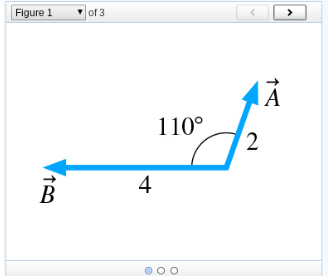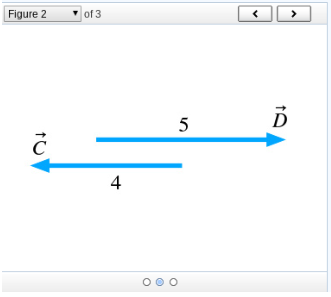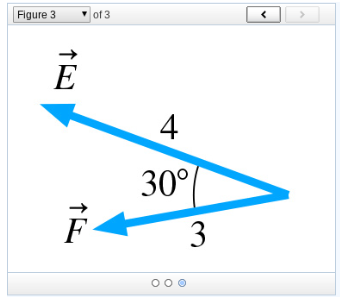# Problem: Part AEvaluate the dot product of the vectors in (Figure 1). Express your answer using two significant figures.Part BEvaluate the dot product of the vectors in (Figure 2). Express your answer using two significant figures.Part CEvaluate the dot product of the vectors in (Figure 3). Express your answer using two significant figures.

###### FREE Expert Solution

Dot product:

$\overline{)\stackrel{\mathbf{⇀}}{\mathbf{A}}{\mathbf{•}}\stackrel{\mathbf{⇀}}{\mathbf{B}}{\mathbf{=}}{\mathbf{ABc}}{\mathbf{o}}{\mathbf{s}}{\mathbf{\theta }}}$

Part A

85% (346 ratings)###### Problem Details

Part A

Evaluate the dot product of the vectors in (Figure 1). Express your answer using two significant figures.Part B

Evaluate the dot product of the vectors in (Figure 2). Express your answer using two significant figures.Part C

Evaluate the dot product of the vectors in (Figure 3). Express your answer using two significant figures.